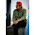## Search This Blog

% Gauss-Seidel method

n=input('Enter number of equations, n:  ');
A = zeros(n,n+1);
x1 = zeros(n);
tol = input('Enter the tolerance, tol: ');
m = input('Enter maximum number of iterations, m:  ');

A=[4 2 3 8; 3 -5 2 -14; -2 3 8 27];
x1=[0 0 0];

k = 1;
while  k <= m
err = 0;
for i = 1 : n
s = 0;
for j = 1 : n
s = s-A(i,j)*x1(j);
end
s = (s+A(i,n+1))/A(i,i);
if abs(s) > err
err  = abs(s);
end
x1(i) = x1(i) + s;
end

if err <= tol
break;
else
k = k+1;
end
end

fprintf('Solution vector after %d iterations is :\n', k-1);
for i = 1 : n
fprintf(' %11.8f \n', x1(i));
end
%Gauss_Seidel.m

%Displaying Gauss_Seidel.m.
OUTPUT:
>> GaussSeidelmethod
Enter number of equations, n:  3
Enter the tolerance, tol: 0.001
Enter maximum number of iterations, m:  100
Solution vector after 8 iterations is :
-0.99984713
3.00008152
2.00000765
>>

1.2.3.MATLAB